### Programming a simple neural network

Though neural networks were considered to be of little use for a long time, the recent development of computing power and database size has proven otherwise. Since the revolution of machine learning in the last few years has been primarily driven by them, let’s dive right into the actual coding of neural nets.

Before coding, it can be useful to review the principles of neural nets to make sure we understand what we will be doing here. Thankfully, the work of coding has already been done by Milo Spencer-Harper, based upon the previous works of Andrew Trask. He guides us step by step into building a single-layer neural net and multiple-layer neural net, with crystal-clear coding and without using any machine learning library.

Since a single-layer neural net is of little use and the problem it solves can better be achieved through other methods, it is included here as a didactic step to better understand and learn to code multiple-layer neural nets.

In these two example, the complexity of the problem, and therefore the number of layers needed to solved it, consists in the number of columns of data to be taken into account. The single layer neural network solves the problem when one column of data is critical, the multiple-layers neural net (2 layers) when two columns are critical.

## Single-layer neural net

In the first post, the building of a simple neural network is detailed through the following key steps synthesized here. The data set is a 3 columns matrix where only one column affects the results.  The single layer neural net is used to understand the direct influence this single column of data over the result.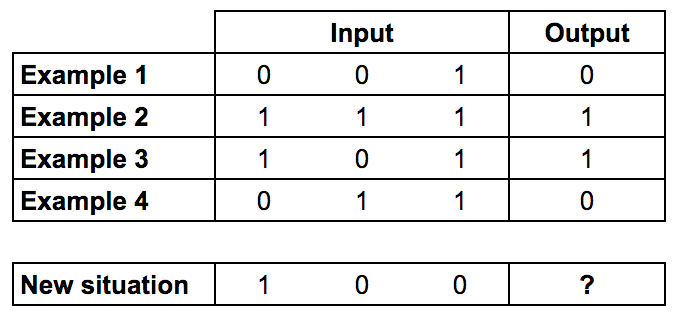Training data: Only the first column of input impacts the output

Since the code has been written in a previous version of Python, here are also included fully functional updated version for Python 3.6. Here is the light 9-line initial code for the single layer neural network.

### Training Process

1. Take inputs, adjust with weights (positive or negative numbers), norm them through a sigmoid function
2. Calculate the error between the neuron’s output and the actual training data set
3. Adjust weights according to the error
4. Repeat 10,000 times

### Calculate the neuron’s output

Sum the weighted input data into a sigmoid function to obtain normed results in the interval ]0;1[The final formula for the output of the neuron is: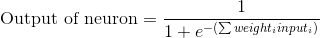Use the error weighted derivative = gradient descent

1. The neuron output from the sigmoid function indicates that if the output is close to 0 or 1, the data was close to the expected result
2. Close to 0 or 1, the derivative of the sigmoid function is almost flat
3. The adjustment to the weights based upon the derivative from the sigmoid function will therefore be very small when the neuron’s output is close to expected results or large when results differ from expectations

The sigmoid derivative equation is: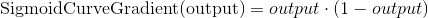So the final equation to adjust weights is: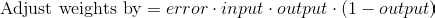Here is a fully functional version of the final code for the single-layer neural network with all details and comments, updated for Python 3.6.

## Multiple-layer neural net

In the second post, the building of a multiple neural network is detailed through the following key steps reproduced. Bear in mind that such a neural network may be to complicated to solve simple problems and that it is best to understand nonlinear patterns, where the second layer of neurons can take combination of data inputs into account.

Here this two-layer neural net is used to understand how two columns in the data influence the results.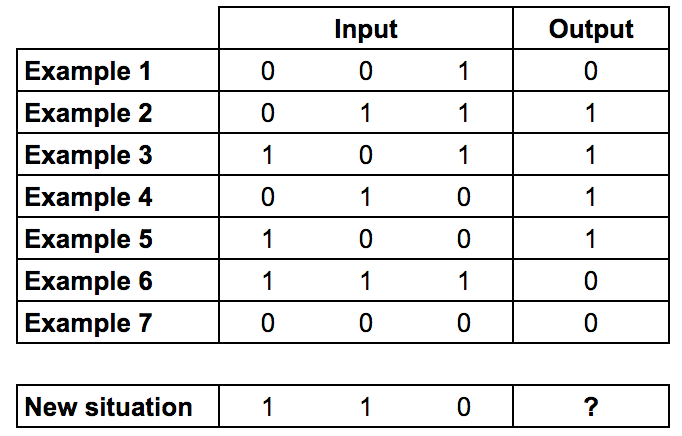Training data: The two first columns of input impact the output (XOR gate)

As Milo Spencer-Harper reminds us in his article, multiple layers are the source of the revolution in machine learning and artificial intelligence:

The process of adding more layers to a neural network, so it can think about combinations, is called deep learning.

The main difference in the code from the single-layer neural net is that the two layers influence the calculations for the error, and therefore the adjustment of weights. The errors from the second layer of neurons need to be propagated backwards to the first layer, this is called backpropagation.

Here is a fully functional version of the code for a two-layer neural network with all details and comments, updated for Python 3.6.

To sum up, in the following video, Siraj Raval goes over the detailed programming of a similar neural net (the original from Andrew Trask) in 4 minutes.

Attention: he uses an older version of Python, he also builds a 3-layer neural net, but the first layer is actually the input data without computation.

## Programming Language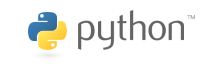Though several programming languages can be used to develop Artificial Intelligence applications, the language that is most often used and advised to use is Python, because it is a general purpose language designed for readability.

### Learning Python

Documentation and resources can be found to use and learn the language on the official Python website, especially with the complete tutorial available for each version of Python.

If you already have programming experience, you can have a brief overview of the syntax and specificity of Python in this video. This great course on problem-solving with algorithms and data structures will give you a complete, step-by-step approach to learning Python with explanations, videos and exercises, while this other tutorial from Stanford quickly goes over Python basics but dives deeper into using the Numpy, SciPy and Matplotlib modules for scientific computing.

Other languages that are often used include: Java, Lisp, Prolog, C++, R…

### Python Programming Tools

Here is a series of programming tools and other systems to assist in the programming of AI applications.

• PyCharm: an Integrated Development Environment – IDE – used in computer programming, specifically for the Python language.
• Jupyter: the Jupyter Notebook is an open-source web application to create and share documents with live code, equations, visualizations.
• NumPy is the fundamental package for scientific computing with Python.
• TensorFlow: an open-source machine learning framework originally developed by Google Brain researchers and engineers.
• Anaconda: Anaconda is a platform for Python and R data science and machine learning on Linux, Windows, and Mac OS X.
• TFLearn: a deep learning library featuring a higher-level API for TensorFlow
• Scikit-Learn: an open-source machine learning library for Python
• Pandas: open-source data structure and analysis library for Python
• Caffe: a deep learning framework made with expression, speed, and modularity in mind
• Keras: a high-level neural networks API, written in Python
• Pytorch: an open source machine learning library for Python, used for applications such as natural language processing.

## Data

List of datasets used for Machine Learning research – Wikipedia

## Cloud services providers

Certain AI applications may require large computing power to process important amounts of data. Fortunately, several companies provide such services on a regular or on-demand basis. Here are the most famous cloud computing services.

## Robotics

ROS – the Construct: Robots and environment simulator to train robots

## Bayesian Story Merging

By using the probability model discovery previously studied, certain concepts and ideas can be analyzed and merged if similar.

## Cross-Modal Coupling

By analyzing the correspondences between clusters of two sets of data, certain data subsets regular correspondences can be sorted out. According to Prof. Patrick Winston, this system of correspondences is very likely to be present in human intelligence.

## Applications of AI

• Scientific approach: understanding how AI works
• Engineering approach: implementing AI applications

The most interesting applications are not to replace people with artificial intelligence but to work in tandem with people.

Using a lot of computing power and data becomes more common, but an interesting question is how little information is needed to work a certain problem.

## Genesis system

The system translates stories into a internal language to understand stories and display them in diagrams. It allows to read stories on different levels, and use different “personas” to understand stories differently.

Humans may not be intelligent enough to build a machine that is as intelligent as them.

## Beliefs nets

Continued from previous class

Events diagrams must always be arranged in a way so that there are final nodes and no loops. Recording probabilities in tables for each event, the tables are filled by repeating experience so as to know the probabilities and occurrences of each event.

### Bayesian inference

Several models can be drawn for a given set of events. To know which model is right, the Bayesian probabilities formulas can be used to confirm if events are independent or not, make them easier to compute, and choose the more appropriate model.

• P(a/b) = P(a,b) / P(b)
• P(a/b) P(b) = P(a,b) = P(b/a) P(a)
• P(a/b) = P(b/a) P(a) / P(b)

Defining a as a class, and b as the evidence, the probability of the evidence given the class can be obtained through these formulas.

P(class/evidence) = P(evidence/class) P(class) / P(evidence)

Using the evidence from experience, classes can inferred by analyzing the results and corresponding probabilities.

### Structure discovery

Given the data from experience / simulation, the right model can be sorted as it better corresponds to the probabilities. This allows to select between 2 existing models.

However if multiple models can be created, volumes of data make it impossible to compare them all. The solution is to use two models and compare them recursively. At each trial, the losing model is modified for improvements until a model fits certain criteria for success.

A trick is to use the sum of the logarithms rather than the probabilities, as large numbers of trials will make numbers too small to compute properly.

To avoid local maxima, a radical rearrangement of structure is launched after a certain number of trials.

### Applications

This Bayesian structure discovery works quite well in situations when a diagnosis must be completed: medical diagnosis, lie-detector, symptoms of aircraft or program not working…